# Mean State

Period Mean (original grids) [W/m2]
Model Period Mean (intersection) [W/m2]
Model Period Mean (complement) [W/m2]
Benchmark Period Mean (intersection) [W/m2]
Benchmark Period Mean (complement) [W/m2]
Bias [W/m2]
RMSE [W/m2]
Phase Shift [months]
Bias Score 
RMSE Score 
Seasonal Cycle Score 
Spatial Distribution Score 
Interannual Variability Score 
Overall Score 
Benchmark [-] 31.3
CLM5PHSOFF [-] 34.7 34.4 32.3 12.4 2.23 8.02 1.94 0.371 0.317 0.717 0.927 0.715 0.561
CLM5PHSON [-] 33.7 33.4 32.3 12.4 1.78 7.73 1.92 0.394 0.331 0.721 0.925 0.696 0.566
Period Mean (original grids) [W/m2]
Model Period Mean (intersection) [W/m2]
Model Period Mean (complement) [W/m2]
Benchmark Period Mean (intersection) [W/m2]
Benchmark Period Mean (complement) [W/m2]
Bias [W/m2]
RMSE [W/m2]
Phase Shift [months]
Bias Score 
RMSE Score 
Seasonal Cycle Score 
Spatial Distribution Score 
Interannual Variability Score 
Overall Score 
Benchmark [-] 44.7
CLM5PHSOFF [-] 46.3 46.8 45.1 13.9 1.52 9.10 0.947 0.526 0.320 0.884 0.980 0.767 0.633
CLM5PHSON [-] 45.2 45.7 45.1 13.9 0.386 8.71 0.894 0.533 0.344 0.891 0.972 0.720 0.634
Period Mean (original grids) [W/m2]
Model Period Mean (intersection) [W/m2]
Model Period Mean (complement) [W/m2]
Benchmark Period Mean (intersection) [W/m2]
Benchmark Period Mean (complement) [W/m2]
Bias [W/m2]
RMSE [W/m2]
Phase Shift [months]
Bias Score 
RMSE Score 
Seasonal Cycle Score 
Spatial Distribution Score 
Interannual Variability Score 
Overall Score 
Benchmark [-] 33.4
CLM5PHSOFF [-] 36.8 36.8 34.3 15.0 2.44 9.00 1.51 0.558 0.376 0.792 0.925 0.750 0.630
CLM5PHSON [-] 36.3 36.3 34.3 15.0 2.16 8.43 1.35 0.555 0.413 0.815 0.902 0.712 0.635
Period Mean (original grids) [W/m2]
Model Period Mean (intersection) [W/m2]
Model Period Mean (complement) [W/m2]
Benchmark Period Mean (intersection) [W/m2]
Benchmark Period Mean (complement) [W/m2]
Bias [W/m2]
RMSE [W/m2]
Phase Shift [months]
Bias Score 
RMSE Score 
Seasonal Cycle Score 
Spatial Distribution Score 
Interannual Variability Score 
Overall Score 
Benchmark [-] 40.8
CLM5PHSOFF [-] 36.9 36.9 41.2 35.6 -4.39 21.6 0.260 0.831 0.662 0.983 0.960 0.667 0.794
CLM5PHSON [-] 33.3 33.3 41.2 35.6 -7.13 23.8 0.214 0.810 0.637 0.986 0.925 0.656 0.775
Period Mean (original grids) [W/m2]
Model Period Mean (intersection) [W/m2]
Model Period Mean (complement) [W/m2]
Benchmark Period Mean (intersection) [W/m2]
Benchmark Period Mean (complement) [W/m2]
Bias [W/m2]
RMSE [W/m2]
Phase Shift [months]
Bias Score 
RMSE Score 
Seasonal Cycle Score 
Spatial Distribution Score 
Interannual Variability Score 
Overall Score 
Benchmark [-] 17.7
CLM5PHSOFF [-] 28.7 28.6 19.3 12.3 9.27 10.8 1.52 0.270 0.357 0.768 0.426 0.694 0.479
CLM5PHSON [-] 27.5 27.5 19.3 12.3 8.88 10.5 1.50 0.280 0.368 0.770 0.361 0.693 0.473
Period Mean (original grids) [W/m2]
Model Period Mean (intersection) [W/m2]
Model Period Mean (complement) [W/m2]
Benchmark Period Mean (intersection) [W/m2]
Benchmark Period Mean (complement) [W/m2]
Bias [W/m2]
RMSE [W/m2]
Phase Shift [months]
Bias Score 
RMSE Score 
Seasonal Cycle Score 
Spatial Distribution Score 
Interannual Variability Score 
Overall Score 
Benchmark [-] 28.1
CLM5PHSOFF [-] 29.5 29.5 28.2 21.3 1.27 12.9 0.212 0.775 0.585 0.986 0.986 0.737 0.776
CLM5PHSON [-] 25.2 25.1 28.2 21.3 -3.04 11.8 0.270 0.789 0.621 0.982 0.977 0.695 0.781
Period Mean (original grids) [W/m2]
Model Period Mean (intersection) [W/m2]
Model Period Mean (complement) [W/m2]
Benchmark Period Mean (intersection) [W/m2]
Benchmark Period Mean (complement) [W/m2]
Bias [W/m2]
RMSE [W/m2]
Phase Shift [months]
Bias Score 
RMSE Score 
Seasonal Cycle Score 
Spatial Distribution Score 
Interannual Variability Score 
Overall Score 
Benchmark [-] 35.8
CLM5PHSOFF [-] 35.0 35.0 36.5 15.2 -1.53 11.1 0.929 0.686 0.539 0.879 0.885 0.694 0.703
CLM5PHSON [-] 35.1 35.1 36.5 15.2 -2.09 11.0 0.920 0.688 0.543 0.883 0.887 0.684 0.705
Period Mean (original grids) [W/m2]
Model Period Mean (intersection) [W/m2]
Model Period Mean (complement) [W/m2]
Benchmark Period Mean (intersection) [W/m2]
Benchmark Period Mean (complement) [W/m2]
Bias [W/m2]
RMSE [W/m2]
Phase Shift [months]
Bias Score 
RMSE Score 
Seasonal Cycle Score 
Spatial Distribution Score 
Interannual Variability Score 
Overall Score 
Benchmark [-] 29.2
CLM5PHSOFF [-] 33.3 33.2 30.4 12.7 2.70 9.72 1.13 0.541 0.415 0.867 0.817 0.703 0.626
CLM5PHSON [-] 33.2 33.2 30.4 12.7 2.27 9.43 1.13 0.551 0.431 0.866 0.800 0.671 0.625
Period Mean (original grids) [W/m2]
Model Period Mean (intersection) [W/m2]
Model Period Mean (complement) [W/m2]
Benchmark Period Mean (intersection) [W/m2]
Benchmark Period Mean (complement) [W/m2]
Bias [W/m2]
RMSE [W/m2]
Phase Shift [months]
Bias Score 
RMSE Score 
Seasonal Cycle Score 
Spatial Distribution Score 
Interannual Variability Score 
Overall Score 
Benchmark [-] 36.1
CLM5PHSOFF [-] 32.7 32.5 36.1 36.1 -3.50 19.6 0.174 0.868 0.682 0.988 0.862 0.698 0.797
CLM5PHSON [-] 31.4 31.3 36.1 36.1 -4.28 20.1 0.202 0.869 0.678 0.986 0.863 0.703 0.796
Period Mean (original grids) [W/m2]
Model Period Mean (intersection) [W/m2]
Model Period Mean (complement) [W/m2]
Benchmark Period Mean (intersection) [W/m2]
Benchmark Period Mean (complement) [W/m2]
Bias [W/m2]
RMSE [W/m2]
Phase Shift [months]
Bias Score 
RMSE Score 
Seasonal Cycle Score 
Spatial Distribution Score 
Interannual Variability Score 
Overall Score 
Benchmark [-] 36.6
CLM5PHSOFF [-] 39.1 39.2 38.5 12.8 0.755 9.14 0.682 0.619 0.559 0.931 0.824 0.707 0.700
CLM5PHSON [-] 39.4 39.4 38.5 12.8 0.0558 9.10 0.622 0.616 0.565 0.942 0.799 0.693 0.696
Period Mean (original grids) [W/m2]
Model Period Mean (intersection) [W/m2]
Model Period Mean (complement) [W/m2]
Benchmark Period Mean (intersection) [W/m2]
Benchmark Period Mean (complement) [W/m2]
Bias [W/m2]
RMSE [W/m2]
Phase Shift [months]
Bias Score 
RMSE Score 
Seasonal Cycle Score 
Spatial Distribution Score 
Interannual Variability Score 
Overall Score 
Benchmark [-] 19.6
CLM5PHSOFF [-] 22.7 22.7 20.5 10.1 2.16 9.06 0.916 0.697 0.557 0.876 0.872 0.681 0.707
CLM5PHSON [-] 22.3 22.3 20.5 10.1 1.68 8.97 0.854 0.691 0.559 0.896 0.842 0.668 0.703
Period Mean (original grids) [W/m2]
Model Period Mean (intersection) [W/m2]
Model Period Mean (complement) [W/m2]
Benchmark Period Mean (intersection) [W/m2]
Benchmark Period Mean (complement) [W/m2]
Bias [W/m2]
RMSE [W/m2]
Phase Shift [months]
Bias Score 
RMSE Score 
Seasonal Cycle Score 
Spatial Distribution Score 
Interannual Variability Score 
Overall Score 
Benchmark [-] 24.0
CLM5PHSOFF [-] 44.9 44.9 44.8 14.0 0.0948 12.9 0.792 0.651 0.538 0.901 0.990 0.704 0.720
CLM5PHSON [-] 44.9 44.9 44.8 14.0 -0.748 12.7 0.768 0.650 0.548 0.906 0.990 0.686 0.722
Period Mean (original grids) [W/m2]
Model Period Mean (intersection) [W/m2]
Model Period Mean (complement) [W/m2]
Benchmark Period Mean (intersection) [W/m2]
Benchmark Period Mean (complement) [W/m2]
Bias [W/m2]
RMSE [W/m2]
Phase Shift [months]
Bias Score 
RMSE Score 
Seasonal Cycle Score 
Spatial Distribution Score 
Interannual Variability Score 
Overall Score 
Benchmark [-] 72.5
CLM5PHSOFF [-] 76.8 76.7 74.7 16.4 1.93 13.9 0.733 0.593 0.558 0.927 0.979 0.710 0.721
CLM5PHSON [-] 78.5 78.3 74.7 16.4 1.75 14.0 0.756 0.590 0.558 0.923 0.980 0.705 0.719
Period Mean (original grids) [W/m2]
Model Period Mean (intersection) [W/m2]
Model Period Mean (complement) [W/m2]
Benchmark Period Mean (intersection) [W/m2]
Benchmark Period Mean (complement) [W/m2]
Bias [W/m2]
RMSE [W/m2]
Phase Shift [months]
Bias Score 
RMSE Score 
Seasonal Cycle Score 
Spatial Distribution Score 
Interannual Variability Score 
Overall Score 
Benchmark [-] 46.1
CLM5PHSOFF [-] 39.5 39.5 46.3 43.2 -6.74 22.9 0.180 0.837 0.671 0.986 0.924 0.680 0.795
CLM5PHSON [-] 37.3 37.3 46.3 43.2 -7.82 24.1 0.184 0.830 0.656 0.986 0.956 0.672 0.793
Period Mean (original grids) [W/m2]
Model Period Mean (intersection) [W/m2]
Model Period Mean (complement) [W/m2]
Benchmark Period Mean (intersection) [W/m2]
Benchmark Period Mean (complement) [W/m2]
Bias [W/m2]
RMSE [W/m2]
Phase Shift [months]
Bias Score 
RMSE Score 
Seasonal Cycle Score 
Spatial Distribution Score 
Interannual Variability Score 
Overall Score 
Benchmark [-] 26.3
CLM5PHSOFF [-] 34.1 34.1 28.9 13.2 5.01 11.1 0.814 0.430 0.467 0.899 0.641 0.710 0.602
CLM5PHSON [-] 33.9 33.9 28.9 13.2 4.55 10.7 0.760 0.435 0.490 0.907 0.620 0.695 0.606
Period Mean (original grids) [W/m2]
Model Period Mean (intersection) [W/m2]
Model Period Mean (complement) [W/m2]
Benchmark Period Mean (intersection) [W/m2]
Benchmark Period Mean (complement) [W/m2]
Bias [W/m2]
RMSE [W/m2]
Phase Shift [months]
Bias Score 
RMSE Score 
Seasonal Cycle Score 
Spatial Distribution Score 
Interannual Variability Score 
Overall Score 
Benchmark [-] 26.9
CLM5PHSOFF [-] 28.4 28.4 27.6 12.3 0.754 8.91 1.21 0.707 0.524 0.818 0.879 0.680 0.689
CLM5PHSON [-] 27.6 27.5 27.6 12.3 -0.305 8.02 1.11 0.718 0.551 0.839 0.834 0.673 0.694
Period Mean (original grids) [W/m2]
Model Period Mean (intersection) [W/m2]
Model Period Mean (complement) [W/m2]
Benchmark Period Mean (intersection) [W/m2]
Benchmark Period Mean (complement) [W/m2]
Bias [W/m2]
RMSE [W/m2]
Phase Shift [months]
Bias Score 
RMSE Score 
Seasonal Cycle Score 
Spatial Distribution Score 
Interannual Variability Score 
Overall Score 
Benchmark [-] 26.3
CLM5PHSOFF [-] 27.0 27.0 26.9 10.9 0.228 13.8 0.325 0.689 0.536 0.962 0.977 0.664 0.727
CLM5PHSON [-] 23.6 23.7 26.9 10.9 -3.27 12.6 0.410 0.720 0.564 0.955 0.970 0.611 0.731
Period Mean (original grids) [W/m2]
Model Period Mean (intersection) [W/m2]
Model Period Mean (complement) [W/m2]
Benchmark Period Mean (intersection) [W/m2]
Benchmark Period Mean (complement) [W/m2]
Bias [W/m2]
RMSE [W/m2]
Phase Shift [months]
Bias Score 
RMSE Score 
Seasonal Cycle Score 
Spatial Distribution Score 
Interannual Variability Score 
Overall Score 
Benchmark [-] 38.3
CLM5PHSOFF [-] 36.1 36.0 38.7 12.8 -2.80 10.1 0.977 0.730 0.566 0.856 0.959 0.755 0.738
CLM5PHSON [-] 34.6 34.6 38.7 12.8 -4.44 10.2 1.05 0.683 0.577 0.846 0.967 0.725 0.729
Period Mean (original grids) [W/m2]
Model Period Mean (intersection) [W/m2]
Model Period Mean (complement) [W/m2]
Benchmark Period Mean (intersection) [W/m2]
Benchmark Period Mean (complement) [W/m2]
Bias [W/m2]
RMSE [W/m2]
Phase Shift [months]
Bias Score 
RMSE Score 
Seasonal Cycle Score 
Spatial Distribution Score 
Interannual Variability Score 
Overall Score 
Benchmark [-] 27.6
CLM5PHSOFF [-] 27.6 27.6 28.0 11.7 -0.361 5.13 1.19 0.567 0.438 0.852 0.808 0.651 0.626
CLM5PHSON [-] 27.1 27.1 28.0 11.7 -0.726 5.09 1.15 0.546 0.448 0.858 0.746 0.627 0.612
Period Mean (original grids) [W/m2]
Model Period Mean (intersection) [W/m2]
Model Period Mean (complement) [W/m2]
Benchmark Period Mean (intersection) [W/m2]
Benchmark Period Mean (complement) [W/m2]
Bias [W/m2]
RMSE [W/m2]
Phase Shift [months]
Bias Score 
RMSE Score 
Seasonal Cycle Score 
Spatial Distribution Score 
Interannual Variability Score 
Overall Score 
Benchmark [-] 31.2
CLM5PHSOFF [-] 32.2 32.2 31.8 11.7 0.390 6.69 1.27 0.695 0.470 0.828 0.881 0.773 0.686
CLM5PHSON [-] 31.6 31.6 31.8 11.7 -0.285 6.44 1.08 0.672 0.494 0.855 0.802 0.725 0.674
Period Mean (original grids) [W/m2]
Model Period Mean (intersection) [W/m2]
Model Period Mean (complement) [W/m2]
Benchmark Period Mean (intersection) [W/m2]
Benchmark Period Mean (complement) [W/m2]
Bias [W/m2]
RMSE [W/m2]
Phase Shift [months]
Bias Score 
RMSE Score 
Seasonal Cycle Score 
Spatial Distribution Score 
Interannual Variability Score 
Overall Score 
Benchmark [-] 30.1
CLM5PHSOFF [-] 32.4 32.3 32.0 13.9 0.318 9.95 0.845 0.561 0.525 0.906 0.963 0.719 0.700
CLM5PHSON [-] 33.2 33.2 32.0 13.9 0.808 9.75 0.826 0.568 0.532 0.908 0.961 0.697 0.700

# Temporally integrated period mean

BENCHMARK MEAN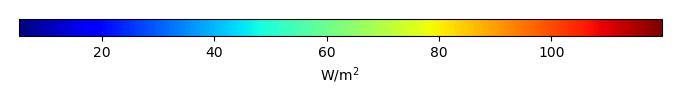MODEL MEANBIAS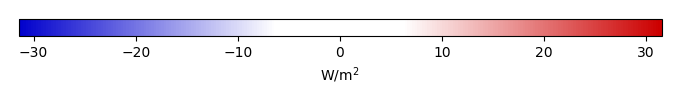BIAS SCORERMSE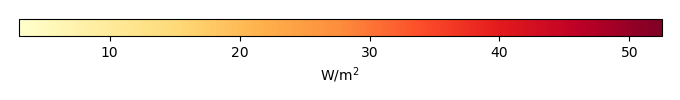RMSE SCOREBENCHMARK INTERANNUAL VARIABILITY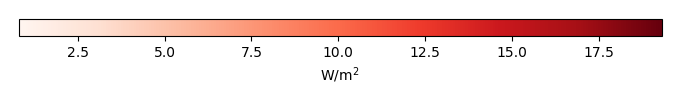MODEL INTERANNUAL VARIABILITYINTERANNUAL VARIABILITY SCOREBENCHMARK MAX MONTHMODEL MAX MONTHDIFFERENCE IN MAX MONTH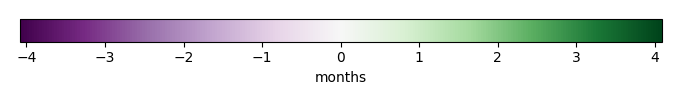SEASONAL CYCLE SCORESPATIAL TAYLOR DIAGRAMMODEL COLORS# Spatially integrated regional mean

MODEL COLORSREGIONAL MEANANNUAL CYCLEMONTHLY ANOMALYANNUAL CYCLE# All Models

BenchmarkCLM5PHSOFFCLM5PHSON# Data Information

creation_date: Thu May 8 23:27:16 PDT 2014

source_file: This product is generated from monthly 1 degree CERES EBAF Radiation observations